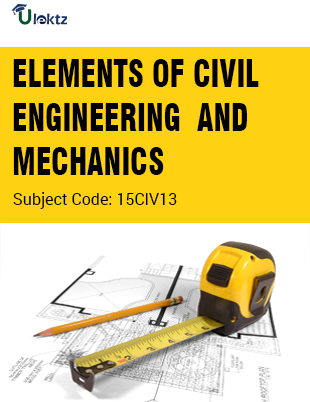•My WalletMy Order
•My Profile
•My Connections
•My Books
•My Videos
•My Tests
•My Calender
•My Messages
•My Shopping Cart
•My Orders
•Account Settings
•Help

# Book Details# Elements of Civil Engineering and Mechanics

 Course Code : 15CIV13 Author : uLektz University : Visvesvaraya Technological University, Karnataka (VTU) Regulation : 2015 Categories : Civil Format :ePUB3 (DRM Protected) Type : eBook

FREE

Description :Elements of Civil Engineering and Mechanics of 15CIV13 covers the latest syllabus prescribed by Visvesvaraya Technological University, Karnataka (VTU) for regulation 2015. Author: uLektz, Published by uLektz Learning Solutions Private Limited.

Note : No printed book. Only ebook. Access eBook using uLektz apps for Android, iOS and Windows Desktop PC.

##### Topics
###### Module 1: Introduction to Civil Engineering &Engineering Mechanics

1.1 Introduction to Civil Engineering-Scope of different fields of Civil Engineering -Surveying, Building Materials, Construction Technology, Geotechnical Engineering, Structural Engineering, Hydraulics, Water Resources and Irrigation Engineering, Transportation Engineering, Environmental Engineering.

1.2 Infrastructure: Types of infrastructure, Role of Civil Engineer in the Infrastructural Development, Effect of the infrastructural facilities on socio-economic development of a country

1.3 Roads: Classification of Roads and their functions, Comparison of Flexible and Rigid Pavements (Advantages and Limitations)

1.4 Bridges: Types of Bridges and Culverts, RCC, Steel and Composite Bridges

1.5 Dams: Different types of Dams based on Material, Structural behavior and functionality with simple sketches.

1.6 Introduction to Engineering Mechanics:Basic idealizations - Particle, Continuum and Rigid body; Newton's laws of Force and its characteristics, types of forces-Gravity, Lateral and its distribution on surfaces, Classification of force systems, Principle of physical independence, superposition, transmissibility of forces, Introduction to SI units

1.7 Couple, Moment of a couple, Characteristics of couple, Moment of a force, Equivalent force - Couple system; Numerical problems on moment of forces and couples, on equivalent force - couple system.

###### Module 2: Analysis of Concurrent Force Systems

2.1 Concepts: Resultants and Equilibrium -Composition of forces - Definition of Resultant; Composition of coplanar -concurrent force system, Parallelogram Law of forces, Principle of resolved parts; Numerical problems on composition of coplanar concurrent force systems.

2.2 Equilibrium of forces - Definition of Equilibrant; Conditions of static equilibrium for different force systems, Lami's theorem; Numerical problems on equilibrium of coplanar – concurrent and non-concurrent force systems.

2.3 Application- Static Friction in rigid bodies in contact-Types of friction, Laws of static friction, Limiting friction, Angle of friction, angle of repose; Impending motion on horizontal and inclined planes; Numerical Problems on single and two blocks on inclined planes

###### Module - 3 Analysis of Non-Concurrent Force Systems

3.1 Concepts: Resultants and Equilibrium Composition of coplanar - non-concurrent force system, Varignon's principle of moments; Numerical problems on composition of coplanar non-concurrent Force system.

3.2 Application-Support Reaction in beams Types of Loads and Supports

3.3 Statically determinate beams - Numerical problems on support reactions for statically determinate beams with Point load (Normal and inclined) and uniformly distributed and uniformly varying loads and Moments

###### Module 4 Centroids and Moments of Inertia of Engineering Sections:

4.1 Centroids -Introduction to the concept, centroid of line and area, centroid of basic geometrical figures, computing centroid for– T, L, I, Z and full/quadrant circular sections and their built up sections. Numerical problems.

4.2 Moment of Inertia -Introduction to the concept, Radius of gyration, Parallel axis theorem, Perpendicular axis theorem, Moment of Inertia of basic planar figures, computing moment of Inertia for – T, L, I, Z and full/quadrant circular sections and their built up sections. Numerical problems

###### Module 5: Kinematics

5.1 Concepts and Applications-Definitions – Displacement – Average velocity – Instantaneous velocity – Speed – Acceleration - Average acceleration – Variable acceleration – Acceleration due to gravity – Newton’s Laws of Motion.

5.2 Rectilinear Motion-Numerical problems.

5.3 Curvilinear Motion – Super elevation – Projectile Motion – Relative motion – Numerical problems.

5.4 Motion under gravity- Numerical problems

### Related Books